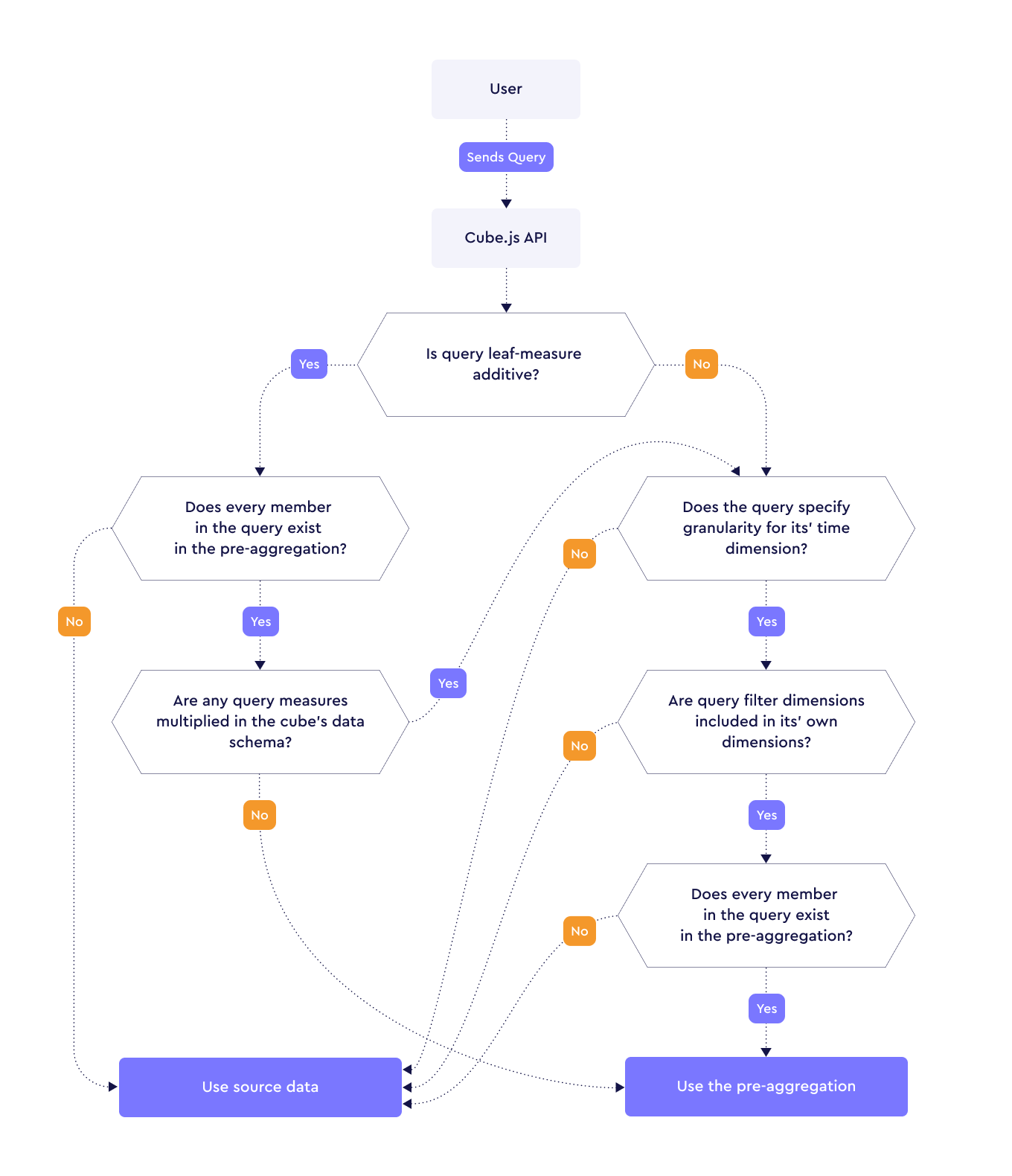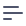# Getting Started with Pre-Aggregations

Often at the beginning of an analytical application's lifecycle - when there is a smaller dataset that queries execute over - the application works well and delivers responses within acceptable thresholds. However, as the size of the dataset grows, the time-to-response from a user's perspective can often suffer quite heavily. This is true of both application and purpose-built data warehousing solutions.

This leaves us with a chicken-and-egg problem; application databases can deliver low-latency responses with small-to-large datasets, but struggle with massive analytical datasets; data warehousing solutions usually make no guarantees except to deliver a response, which means latency can vary wildly on a query-to-query basis.

Database TypeLow Latency?Massive Datasets?
Application (Postgres/MySQL)
Analytical (BigQuery/Redshift)

Cube provides a solution to this problem: pre-aggregations. In layman's terms, a pre-aggregation is a condensed version of the source data. It specifies attributes from the source, which Cube uses to condense (or crunch) the data. This simple yet powerful optimization can reduce the size of the dataset by several orders of magnitude, and ensures subsequent queries can be served by the same condensed dataset if any matching attributes are found.

Pre-aggregations are defined within each cube's data schema, and cubes can have as many pre-aggregations as they require. The pre-aggregated data can be stored either alongside the source data in the same database, in an external database that is supported by Cube, or in Cube Store, a dedicated pre-aggregation storage layer.

## Pre-Aggregations without Time Dimension

To illustrate pre-aggregations with an example, let's use a sample e-commerce database. We have a schema representing all our Orders:

cube(Orders, {
sql: SELECT * FROM public.orders,

measures: {
count: {
type: count,
drillMembers: [id, createdAt],
},
},

dimensions: {
status: {
sql: status,
type: string,
},

id: {
sql: id,
type: number,
primaryKey: true,
},

completedAt: {
sql: completed_at,
type: time,
},
},
});

Some sample data from this table might look like:

idstatuscompleted_at
1completed2021-02-15T12:21:11.290
2completed2021-02-25T18:15:12.369
3shipped2021-03-15T20:40:57.404
4processing2021-03-13T10:30:21.360
5completed2021-03-10T18:25:32.109

Our first requirement is to populate a dropdown in our front-end application which shows all possible statuses. The Cube query to retrieve this information might look something like:

{
"dimensions": ["Orders.status"]
}
cube(Orders, {
// Same content as before, but including the following:
preAggregations: {
orderStatuses: {
dimensions: [status],
},
},
});

## Pre-Aggregations with Time Dimension

Using the same schema as before, we are now finding that users frequently query for the number of orders completed per day, and that this query is performing poorly. This query might look something like:

{
"measures": ["Orders.count"],
"timeDimensions": ["Orders.completedAt"]
}

In order to improve the performance of this query, we can add another pre-aggregation definition to the Orders schema:

cube(Orders, {
// Same content as before, but including the following:
preAggregations: {
ordersByCompletedAt: {
measures: [count],
timeDimension: completedAt,
granularity: month,
},
},
});

Note that we have added a granularity property with a value of month to this definition. This allows Cube to aggregate the dataset to a single entry for each month.

The next time the API receives the same JSON query, Cube will build (if it doesn't already exist) the pre-aggregated dataset, store it in the source database server and use that dataset for any subsequent queries. A sample of the data in this pre-aggregated dataset might look like:

completed_atcount
2021-02-01T00:00:00.0002
2021-03-01T00:00:00.0003

## Keeping pre-aggregations up-to-date

Pre-aggregations can become out-of-date or out-of-sync if the original dataset changes. Cube uses a refresh key to check the freshness of the data; if a change in the refresh key is detected, the pre-aggregations are rebuilt. These refreshes are performed in the background as a scheduled process, unless configured otherwise.

## Ensuring pre-aggregations are targeted by queries

Cube selects the best available pre-aggregation based on the incoming queries it receives via the API. The process for selection is summarized below:

1. Are all measures of type count, sum, min, max or countDistinctApprox?

2. If yes, then check if

3. If no, then check if

• The query's time dimension granularity is set
• All query filter dimensions are included in query dimensions
• The pre-aggregation defines the exact set of dimensions and measures used in the query

You can find a complete flowchart here.

So far, we've described pre-aggregations as aggregated versions of your existing data. However, there are some rules that apply when Cube uses the pre-aggregation. The additivity of fields specified in both the query and in the pre-aggregation determines this.

So what is additivity? Let's add another cube called LineItems to the previous example to demonstrate. The LineItems belong to the Orders cube, and are joined as such:

cube(LineItems, {
sql: SELECT * FROM public.line_items,

joins: {
Orders: {
sql: ${CUBE}.order_id =${Orders.id},
relationship: belongsTo,
},
},

measures: {
count: {
type: count,
drillMembers: [id, createdAt],
},
},

dimensions: {
id: {
sql: id,
type: number,
primaryKey: true,
},

createdAt: {
sql: created_at,
type: time,
},
},
});

Some sample data from the line_items table might look like:

idproduct_idorder_idquantitypriceprofit_margincreated_at
1311127512021-01-20 00:00:00.000000
249262480.12021-01-20 00:00:00.000000
389361970.352021-01-21 00:00:00.000000
471482230.152021-01-21 00:00:00.000000
56455750.752021-01-22 00:00:00.000000
66268750.652021-01-22 00:00:00.000000

Looking at the raw data, we can see that if the data were to be aggregated by created_at, then we could simply add together the quantity and price fields and still get a correct result:

created_atquantityprice
2021-01-20 00:00:00.0000007523
2021-01-21 00:00:00.00000014420
2021-01-22 00:00:00.00000013150

This means that quantity and price are both additive measures, and we can represent them in the LineItems schema as follows:

cube(LineItems, {
...,
measures: {
...,
quantity: {
sql: quantity,
type: sum,
},
price: {
type: sum,
sql: price,
format: currency,
},
},
...,
});

Because neither quantity and price reference any other measures in our LineItems cube, we can also say that they are additive leaf measures. Any query requesting only these two measures can be called a leaf measure additive query. Additive leaf measures can only be of the following types: count, sum, min, max or countDistinctApprox.

Using the same sample data for line_items, there's a profit_margin field which is different for each row. However, despite the value being numerical, it doesn't actually make sense to add up this value. Let's look at the rows for 2021-01-20 in the sample data:

idproduct_idorder_idquantitypriceprofit_margincreated_at
1311127512021-01-20 00:00:00.000000
249262480.12021-01-20 00:00:00.000000

And now let's try and aggregate them:

created_atquantitypriceprofit_margin
2021-01-20 00:00:00.00000075231.1

Using the source data, we'll manually calculate the profit margin and see if it matches the above. We'll use the following formula:

$x + (x * y) = z$

Where x is the original cost of the item, y is the profit margin and z is the price the item was sold for. Let's use the formula to find the original cost for both items sold on 2021-01-20. For the row with id = 1:

$x + (x * 1) = 275\\ 2x = 275\\ x = 275 / 2\\ x = 137.5$

And for the row where id = 2:

$x + (x * 0.1) = 248\\ 1.1x = 248\\ x = 248 / 1.1\\ x = 225.454545454545455$

Which means the total cost for both items was:

$225.454545454545455 + 137.5\\ 362.954545454545455$

Now that we have the cost of each item, let's use the same formula in reverse to see if applying a profit margin of 1.1 will give us the same total price (523) as calculated earlier:

$362.954545454545455 + (362.954545454545455 * 1.1) = z\\ 762.204545454545455 = z\\ z = 762.204545454545455$

We can clearly see that 523 does not equal 762.204545454545455, and we cannot treat the profit_margin column the same as we would any other additive measure. Armed with the above knowledge, we can add the profit_margin field to our schema as a dimension:

cube(LineItems, {
...,
dimensions: {
...,
profitMargin: {
sql: profit_margin,
type: number,
format: 'percentage',
},
},
...,
});

Another approach might be to calculate the profit margin dynamically, and instead saving the "cost" price. Because the cost price is an additive measure, we are able to store it in a pre-aggregation:

cube(LineItems, {
...,
measures: {
...,
cost: {
sql: ${CUBE.price} / (1 +${CUBE.profitMargin}),
type: sum,
},
},
...,
});

Another example of a non-additive measure would be a distinct count of product_id. If we took the distinct count of products sold over a month, and then tried to sum the distinct count of products for each individual day and compared them, we would not get the same results. We can add the measure like this:

cube(LineItems, {
...,
measures: {
...,
countDistinctProducts: {
sql: product_id,
type: countDistinct,
},
},
...,
});

However the above cannot be used in for a pre-aggregation. We can instead change the type to countDistinctApprox, and then use the measure in a pre-aggregation definition:

cube(LineItems, {
...,
measures: {
...,
countDistinctProducts: {
sql: product_id,
type: countDistinctApprox,
},
},
preAggregations: {
myRollup: {
...,
measures: [ CUBE.countDistinctProducts ],
}
},
...,
});

### Selecting the pre-aggregation

To recap what we've learnt so far:

• Multiplied measures are measures that define hasMany relations

• Leaf measures are measures that do not reference any other measures in their definition

• Calculated measures are measures that reference other dimensions and measures in their definition

• A query is leaf measure additive if all of its leaf measures are one of: count, sum, min, max or countDistinctApprox

Cube looks for matching pre-aggregations in the order they are defined in a cube's schema file. Each defined pre-aggregation is then tested for a match based on the criteria in the flowchart below:Some extra considerations for pre-aggregation selection:

• The query's time dimension and granularity must match the pre-aggregation.

• The query's time dimension and granularity together act as a dimension. If the date range isn't aligned with granularity, a common granularity is used. This common granularity is selected using the greatest common divisor across both the query and pre-aggregation. For example, the common granularity between hour and day is hour because both hour and day can be divided by hour.

• The query's granularity's date range must match the start date and end date from the time dimensions. For example, when using a granularity of month, the values should be the start and end days of the month i.e. ['2020-01-01T00:00:00.000', '2020-01-31T23:59:59.999']; when the granularity is day, the values should be the start and end hours of the day i.e. ['2020-01-01T00:00:00.000', '2020-01-01T23:59:59.999']. Date ranges are inclusive, and the minimum granularity is second.

• The order in which pre-aggregations are defined in schemas matter; the first matching pre-aggregation for a query is the one that is used. Both the measures and dimensions of any cubes specified in the query are checked to find a matching rollup.

• rollup pre-aggregations always have priority over originalSql. Thus, if you have both originalSql and rollup defined, Cube will try to match rollup pre-aggregations before trying to match originalSql. You can instruct Cube to use the original SQL pre-aggregations by using useOriginalSqlPreAggregations.